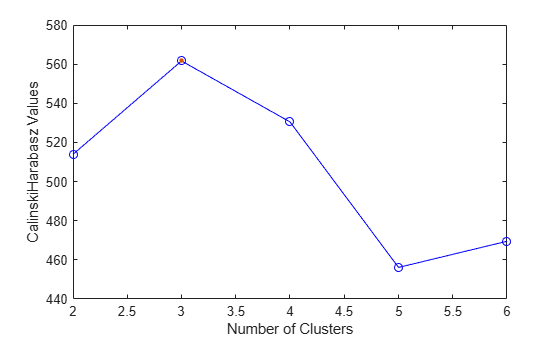Documentation

# CalinskiHarabaszEvaluation

Package: clustering.evaluation
Superclasses: `clustering.evaluation.ClusterCriterion`

Calinski-Harabasz criterion clustering evaluation object

## Description

`CalinskiHarabaszEvaluation` is an object consisting of sample data, clustering data, and Calinski-Harabasz criterion values used to evaluate the optimal number of clusters. Create a Calinski-Harabasz criterion clustering evaluation object using `evalclusters`.

## Construction

`eva = evalclusters(x,clust,'CalinskiHarabasz')` creates a Calinski-Harabasz criterion clustering evaluation object.

`eva = evalclusters(x,clust,'CalinskiHarabasz',Name,Value)` creates a Calinski-Harabasz criterion clustering evaluation object using additional options specified by one or more name-value pair arguments.

### Input Arguments

expand all

Input data, specified as an N-by-P matrix. N is the number of observations, and P is the number of variables.

Data Types: `single` | `double`

Clustering algorithm, specified as one of the following.

 `'kmeans'` Cluster the data in `x` using the `kmeans` clustering algorithm, with `'EmptyAction'` set to `'singleton'` and `'Replicates'` set to `5`. `'linkage'` Cluster the data in `x` using the `clusterdata` agglomerative clustering algorithm, with `'Linkage'` set to `'ward'`. `'gmdistribution'` Cluster the data in `x` using the `gmdistribution` Gaussian mixture distribution algorithm, with `'SharedCov'` set to `true` and `'Replicates'` set to `5`.

If `criterion` is `'CalinskiHarabasz'`, `'DaviesBouldin'`, or `'silhouette'`, you can specify a clustering algorithm using a function handle (MATLAB). The function must be of the form `C = clustfun(DATA,K)`, where `DATA` is the data to be clustered, and `K` is the number of clusters. The output of `clustfun` must be one of the following:

• A vector of integers representing the cluster index for each observation in `DATA`. There must be `K` unique values in this vector.

• A numeric n-by-K matrix of score for n observations and K classes. In this case, the cluster index for each observation is determined by taking the largest score value in each row.

If `criterion` is `'CalinskiHarabasz'`, `'DaviesBouldin'`, or `'silhouette'`, you can also specify `clust` as a n-by-K matrix containing the proposed clustering solutions. n is the number of observations in the sample data, and K is the number of proposed clustering solutions. Column j contains the cluster indices for each of the N points in the jth clustering solution.

Data Types: `single` | `double` | `char` | `string` | `function_handle`

#### Name-Value Pair Arguments

Specify optional comma-separated pairs of `Name,Value` arguments. `Name` is the argument name and `Value` is the corresponding value. `Name` must appear inside quotes. You can specify several name and value pair arguments in any order as `Name1,Value1,...,NameN,ValueN`.

Example: `'KList',[1:6]` specifies to test 1, 2, 3, 4, 5, and 6 clusters to find the optimal number.

List of number of clusters to evaluate, specified as the comma-separated pair consisting of `'KList'` and a vector of positive integer values. You must specify `KList` when `clust` is a clustering algorithm name or a function handle. When `criterion` is `'gap'`, `clust` must be a character vector, a string scalar, or a function handle, and you must specify `KList`.

Example: `'KList',[1:6]`

Data Types: `single` | `double`

## Properties

 `ClusteringFunction` Clustering algorithm used to cluster the input data, stored as a valid clustering algorithm name or function handle. If the clustering solutions are provided in the input, `ClusteringFunction` is empty. `CriterionName` Name of the criterion used for clustering evaluation, stored as a valid criterion name. `CriterionValues` Criterion values corresponding to each proposed number of clusters in `InspectedK`, stored as a vector of numerical values. `Distance` Distance metric used for clustering data, stored as a valid distance metric name. `InspectedK` List of the number of proposed clusters for which to compute criterion values, stored as a vector of positive integer values. `Missing` Logical flag for excluded data, stored as a column vector of logical values. If `Missing` equals `true`, then the corresponding value in the data matrix `x` is not used in the clustering solution. `NumObservations` Number of observations in the data matrix `X`, minus the number of missing (`NaN`) values in `X`, stored as a positive integer value. `OptimalK` Optimal number of clusters, stored as a positive integer value. `OptimalY` Optimal clustering solution corresponding to `OptimalK`, stored as a column vector of positive integer values. If the clustering solutions are provided in the input, `OptimalY` is empty. `X` Data used for clustering, stored as a matrix of numerical values.

## Methods

### Inherited Methods

 addK Evaluate additional numbers of clusters compact Compact clustering evaluation object plot Plot clustering evaluation object criterion values

## Examples

collapse all

Evaluate the optimal number of clusters using the Calinski-Harabasz clustering evaluation criterion.

`load fisheriris;`

The data contains length and width measurements from the sepals and petals of three species of iris flowers.

Evaluate the optimal number of clusters using the Calinski-Harabasz criterion. Cluster the data using `kmeans`.

```rng('default'); % For reproducibility eva = evalclusters(meas,'kmeans','CalinskiHarabasz','KList',[1:6])```
```eva = CalinskiHarabaszEvaluation with properties: NumObservations: 150 InspectedK: [1 2 3 4 5 6] CriterionValues: [NaN 513.9245 561.6278 530.4871 456.1279 469.5068] OptimalK: 3 ```

The `OptimalK` value indicates that, based on the Calinski-Harabasz criterion, the optimal number of clusters is three.

Plot the Calinski-Harabasz criterion values for each number of clusters tested.

```figure; plot(eva);```The plot shows that the highest Calinski-Harabasz value occurs at three clusters, suggesting that the optimal number of clusters is three.

Create a grouped scatter plot to examine the relationship between petal length and width. Group the data by suggested clusters.

```PetalLength = meas(:,3); PetalWidth = meas(:,4); ClusterGroup = eva.OptimalY; figure; gscatter(PetalLength,PetalWidth,ClusterGroup,'rbg','xod');```The plot shows cluster 3 in the lower-left corner, completely separated from the other two clusters. Cluster 3 contains flowers with the smallest petal widths and lengths. Cluster 1 is in the upper-right corner, and contains flowers with the largest petal widths and lengths. Cluster 2 is near the center of the plot, and contains flowers with measurements between these two extremes.

expand all

## References

 Calinski, T., and J. Harabasz. “A dendrite method for cluster analysis.” Communications in Statistics. Vol. 3, No. 1, 1974, pp. 1–27.